.boxed { border: 1px solid green ; }

# Using Math in the Physics Classroom Part I: Units and Conversions

By Chris Meyer

Do you shake your fist at the skies when you look over your student’s math work? Do you find yourself cursing the math teachers in your school? If you do, you are likely not alone. But are our grievances and grudges justified? As a physics teacher, it can feel very perplexing to watch one’s students struggle to use grade 10 mathematics in our grade 12 physics classes. Let’s give our math teachers a break for the moment and explore a surprising factor in our physics students’ struggle: the dialect of math that we speak in a physics classroom.

Lost in translation
Math in the physics classroom and math in the math classroom are two distinct dialects of one language. If you have ever traveled to some far, or not so far, flung region of the English-speaking world, you may have experienced the challenge of communicating with the locals in our shared language. I remember when my wife and I visited a park in Glasgow and talked to some children; we were utterly baffled. Or the next time you have a chance, strike up a conversation with a British person and compliment them on the nice pants they are wearing today. Let me know how that goes1. The math community and physics community speak different enough dialects of math that students can easily get lost in translation. You and I have been immersed in both dialects and cultures for many years and have forgotten the very real differences. As a result, we can fail to recognize the challenges our students experience using math in our physics classrooms.

Units as the Final Touch
My students often comment that in math class they are instructed to put in the units at the end of a calculation. Just like that … parachute them in! Math classes use a dialect of math that is optimized to explore abstract quantitative relationships. In that context, units are a final touch and something that should not encumber mathematical work or obscure mathematical relationships. From a math lesson on parabolas, a typical application might be describing the height in metres of a ball tossed upwards through the air: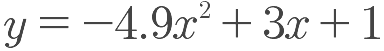This doesn’t look anything like what we do in a physics class. In this mathematical representation, there are no explicit clues that hint at the expression’s physical meaning. It could be describing anything, and that’s actually the point from the math class perspective. The pure mathematical relationships are very clear.

Searching for Meaning
While the “final touch” approach to units is understandable in a math class, in science we need a different strategy. Carrying units through a scientific calculation provides a number of benefits for students. Units can provide a valuable check of their arithmetic or algebraic work. If the units “work out” by the end of a calculation, there is a greater chance that the math work was done correctly.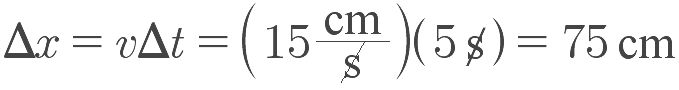Scientific calculations always have a physical meaning. Having units allows students to properly interpret a mathematical result, especially a novel result. For example, a student might be finding the slope of a position graph for the first time: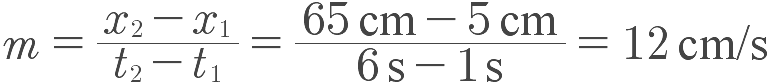Carrying units through the calculation allows students to recognize that the slope represents an object’s speed or velocity. Training students to interpret results like this deepens their understanding. For example, the slope result means: “if the object continues to move with a constant velocity, then for every second that goes by, it would travel 12 cm forward.” Even the awkward seeming gob of units in the quantity (5 km/h)/s provides a deeper understanding of the acceleration concept because it can be carefully interpreted: “for every second that goes by, the velocity changes by +5 km/h). This is only possible when units are present.

Units Change the Meaning of Math Operations
Do you remember how young elementary students are taught to picture the multiplication operation? It’s been a while? The product 3×5 can be pictured as three groups of five things (like three groups of five apples), providing a clear model for discovering the result of 15. It could also be pictured as five groups of three apples. The constant here is that the thing itself does not change: with either method of grouping, we are still picturing apples. But what about this physics calculation: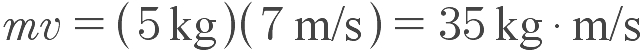Hmm? It looks so innocuous, but conceptually it behaves very differently from the elementary school example! We don’t end up with five groups of seven velocities, or seven groups of five-kilo masses. We end up with a totally different kind of thing. We end up with momentum: one of the few conserved quantities of our classical universe. Neither mass nor velocity have this most wonderful property. While the number part of the calculation obeys the elementary school model’s pattern, the physical meaning is shaped in a dramatic way by the units2.

When Units Collide
Carrying units through a calculation can also provide a valuable warning.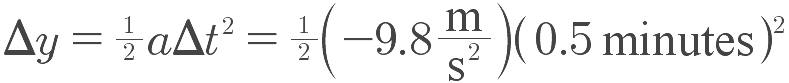Watch out! In this calculation, the units for time do not agree and will yield an erroneous result in metres if the numbers are blindly plugged into a calculator. The careful use of units helps us understand when conversions are necessary, and we can train students to spot this in advance of their math work.

Bungled Conversions
I used to teach conversions as something you just wing (am I the only one?). Find the conversion factor, hopefully the right one, and just use it! No, not 2.2. Use 0.454. Do you multiply or divide it? Roll the dice! My teaching of conversations was very sloppy because I had never cleared up the logic and meaning of the conversion process in my own mind, I just winged it. But I could tell when I was doing it wrong, so I would quickly fix it in my head and show students the right way! If you teach conversions as a strictly mathematical operation, you miss exploring the deeper meaning and logic behind it.

Conversions Don’t Change Anything
The goal of a conversion is not to change anything! Well, anything physically meaningful. After the conversion, the car must still have the same speed, the person the same mass, and the duration until your retirement is the same amount of time (regardless of whether you are counting it in years or seconds). This fact limits our options. If you used to walk to school up-hill in the snow 7 miles, there is only one number we can multiply that epic distance by such that it remains the same: one! And there you have it. Every conversion must be performed by multiplying the original quantity by one. Balderdash, you say? Well, one is a magical number because it can be represented in many ways. When it comes to conversions, one is best represented as a ratio, what I call the conversion ratio.The Conversion Ratio
The conversion ratio is constructed such that the quantities in the numerator and denominator are equal, making the ratio equal to one. How do we determine which quantity should go on the top or bottom? No problem! We want the undesirable units (imperial!) to divide out. Avoid describing this as “canceling out”. Students “cancel out” (and mentally check out) so often in their math classes that they no longer remember what mathematical operation is at work behind “cancelling out”. Just ask them and you’ll see! The conversion ratio strategy provides a consistent logical structure that allows students to decide whether to multiply or divide by 1609. The strategy is robust enough to handle conversions of any complexity: just keep multiplying by one!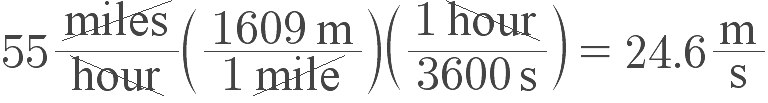Conversion Number Sense
To pull off the conversion calculations above, there is another piece of hidden math that must be brought to light. The original quantity “7 miles” has a hidden operation between the “7” and “miles”. Did you spot it and do you teach it?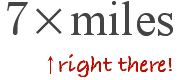This multiplication operation follows our elementary school model of multiplication very nicely. Reminding students of this helps them activate their number sense, which helps them decide whether the converted result seems right. We can measure this impressive distance using a small sized ruler (a metre stick) or using a very large sized ruler (a mile stick!). If we use the small ruler, how many rulers would we need to cover this distance? How about with the bigger ruler? So, if we convert from miles to metres, do we expect the number part to be bigger or smaller? This is a powerful sense-making skill that is worth developing.

Science!
Measurements are the backbone of doing science and all science numbers tell us something about our physical world. Without units, these numbers lose their power to describe phenomena and test ideas. Our experience speaking both dialects of math allows us teachers to forget the challenges our students experience as we casually slip back and forth, like my family’s Frenglish dinner conversations. Units need care and attention in our teaching. Taking that care helps our students to learn deeper and more easily.

Endnotes
1 “pants” in British English means underwear.

2 From a conversation with Eugenia Etkina, October 2016